## Introduction

This is complementary vignette to the paper Helske (2022) with more detailed examples and a short comparison with naive Stan implementation for regression model with time-varying coefficients. The varying coefficient regression models are extension to basic linear regression models where instead of constant but unknown regression coefficients, the underlying coefficients are assumed to vary over time according to random walk. In their simplest form these models can be used to model regression models with additional time series component, and they also allow robust modelling of phenomenas where the effect size of the predictor variables can vary during the period of the study, for example due to interactions with unobserved counfounders. The R package walker provides an efficient method for fully Bayesian inference of such models, where the main computations are performed using the Markov chain Monte Carlo (MCMC) algorithms provided by Stan Stan Development Team (2016a). This also allows the use of many general diagnostic and graphical tools provided by several Stan related R packages such as ShinyStan . the linear model with time-varying coefficients is defined as

A linear model with time-varying coefficients defined as \begin{aligned} y_t &= x'_t \beta_t + \epsilon_t, \quad t = 1,\ldots, n\\ \beta_{t+1} &= \beta_t + \eta_t, \end{aligned} where $$y_t$$ is the observation at time $$t$$, $$x_t$$ contains the corresponding predictor variables, $$\beta_t$$ is a $$k$$ dimensional vector of regression coefficients at time $$t$$, $$\epsilon_t \sim N(0, \sigma^2_{\epsilon})$$, and $$\eta_t \sim N(0, D)$$, with $$D$$ being $$k \times k$$ diagonal matrix with diagonal elements $$\sigma^2_{i,\eta}$$, $$i=1,\ldots,k$$. Denote the unknown parameters of the model by $$\beta = (\beta'_1, \ldots, \beta'_n)'$$ and $$\sigma = (\sigma_{\epsilon}, \sigma_{1, \eta}, \ldots, \sigma_{k, \eta})$$. We define priors for first $$\beta_1$$ as $$N(\mu_{\beta_1}, \sigma_{\beta_1})$$, and for $$\sigma_i \sim N(\mu_{\sigma_i}, \sigma_{\sigma_i})$$, $$i=1,\ldots,k+1$$, truncated to positive values.

Although in principle writing this model as above in Stan is straightforward, standard implementation can lead to some computational problems (see the illustration in next Section). An alternative solution used by walker is based on the marginalization of the regression coefficients $$\beta$$ during the MCMC sampling by using the Kalman filter. This provides fast and accurate inference of marginal posterior $$p(\sigma | y)$$. Then, the corresponding joint posterior $$p(\sigma, \beta | y) = p(\beta | \sigma, y)p(\sigma | y)$$ can be obtained by simulating the regression coefficients given marginal posterior of standard deviations. This sampling can be performed for example by simulation smoothing algorithm by Durbin and Koopman (2002). Note that we have opted to sample the $$\beta$$ parameters given $$\sigma$$’s, but it is also possible to obtain somewhat more accurate summary statistics such as mean and variance of these parameters by using the standard Kalman smoother for computation of $$\textrm{E}(\beta| \sigma, y)$$ and $$\textrm{Var}(\beta| \sigma, y)$$, and using the law of total expectation.

In this vignette we first introduce the basic use using simple linear regression model with first order random walk, and then discuss extensions to second order random walk, as well as how to deal with non-Gaussian data.

## Illustration

Let us consider a observations $$y$$ of length $$n=100$$, generated by random walk (i.e. time varying intercept) and two predictors. This is rather small problem, but it was chosen in order to make possible comparisons with the “naive” Stan implementation. For larger problems (in terms of number of observations and especially number of predictors) it is very difficult to get naive implementation to work properly, as even after tweaking several parameters of the underlying MCMC sampler, one typically ends up with divergent transitions or low BMFI index, meaning that the results are not to be trusted.

First we simulate the coefficients and the predictors:

set.seed(1)
n <- 100
beta1 <- cumsum(c(0.5, rnorm(n - 1, 0, sd = 0.05)))
beta2 <- cumsum(c(-1, rnorm(n - 1, 0, sd = 0.15)))
x1 <- rnorm(n, mean = 2)
x2 <- cos(1:n)
rw <- cumsum(rnorm(n, 0, 0.5))
ts.plot(cbind(rw, beta1 * x1, beta2 * x2), col = 1:3)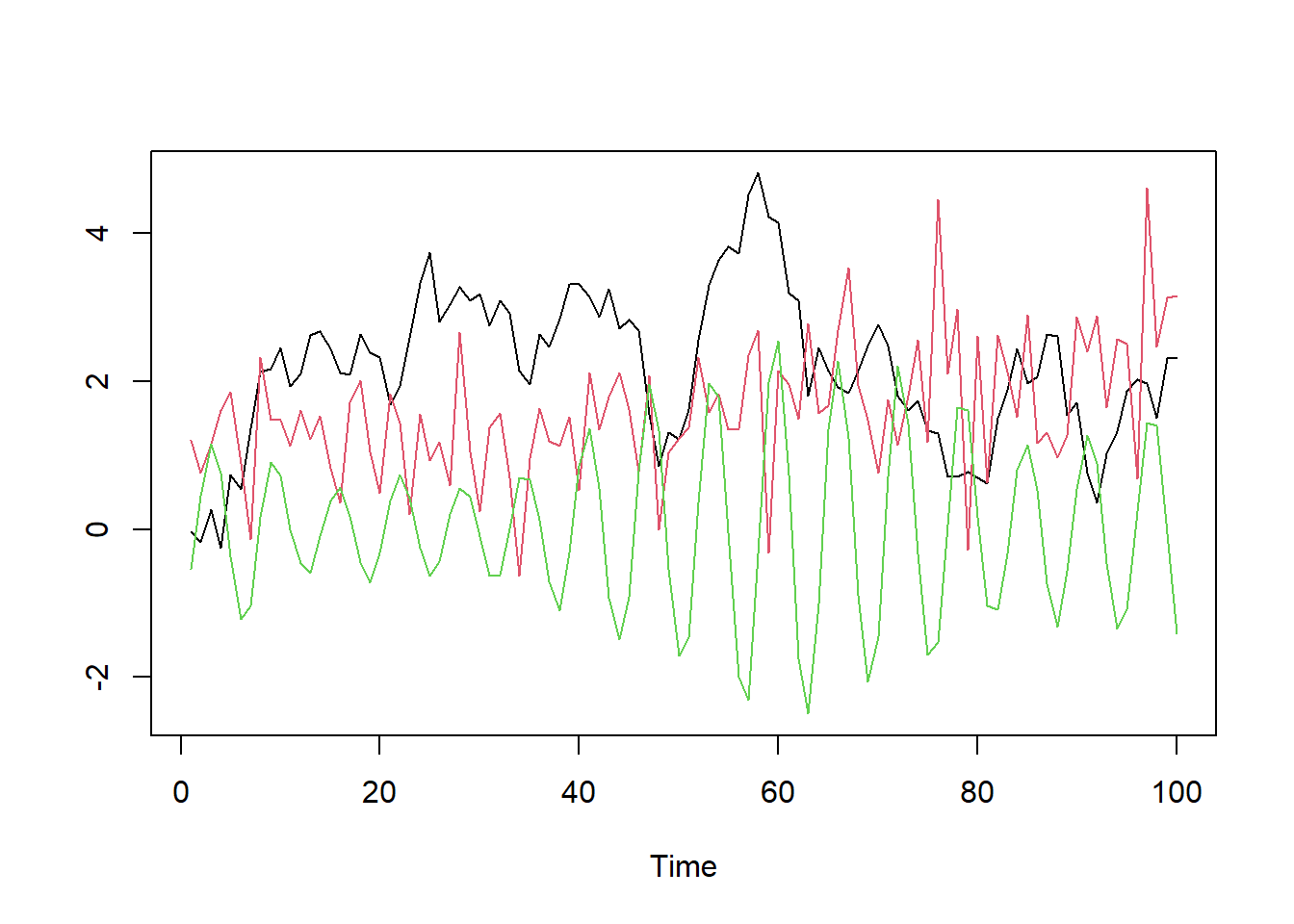signal <- rw + beta1 * x1 + beta2 * x2
y <- rnorm(n, signal, 0.5)
ts.plot(cbind(signal, y), col = 1:2)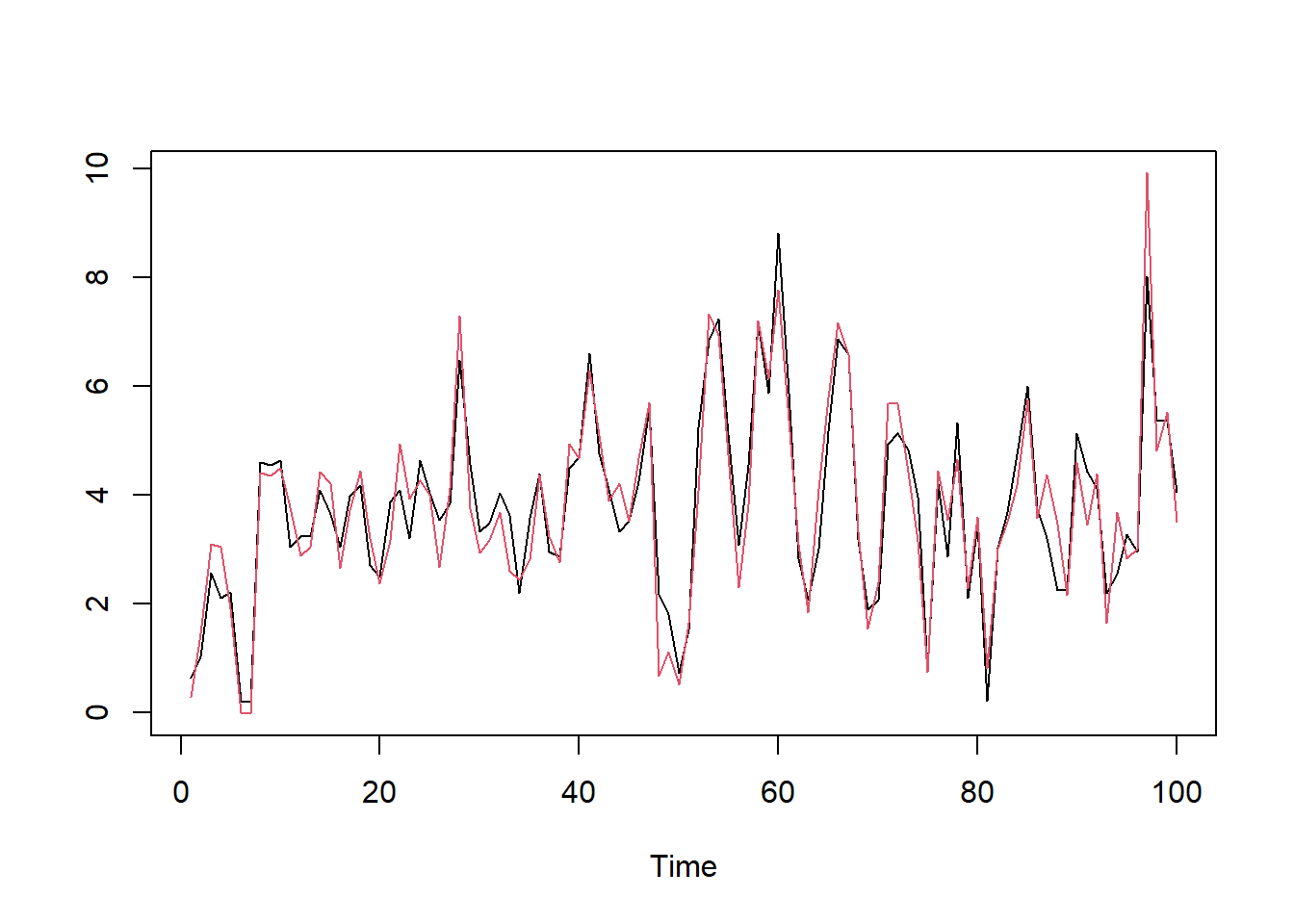Then we can call function walker. The model is defined as a formula like in lm, and we can give several arguments which are passed to sampling method of rstan, such as number of iteration iter and number of chains chains (default values for these are 2000 and 4). In addition to these, we use arguments beta and sigma, which define the Gaussian and Gamma prior distributions for $$\beta_1$$ and $$\sigma$$ respectively. These arguments should be vectors of length two defining the parameters of the prior distributions: mean and sd for the coefficients, and shape and rate for standard deviation parameters).

set.seed(1)
fit <- walker(y ~ -1 + rw1(~ x1 + x2, beta = c(0, 10), sigma = c(2, 10)),
refresh = 0, chains = 1, sigma_y = c(2, 1))

We sometimes get a few (typically one) warning message about numerical problems, as the sampling algorithm warms up, but this is nothing to be concerned with (if more errors occur, then a Github issue for walker package is more than welcome).

The output of walker is walker_fit object, which is essentially a list with stanfit from Stan’s sampling function, and the original observations y and the covariate matrix xreg. Thus we can use all the available tools for postprocessing stanfit objects:

print(fit$stanfit, pars = c("sigma_y", "sigma_rw1")) ## Inference for Stan model: walker_lm. ## 1 chains, each with iter=2000; warmup=1000; thin=1; ## post-warmup draws per chain=1000, total post-warmup draws=1000. ## ## mean se_mean sd 2.5% 25% 50% 75% 97.5% n_eff Rhat ## sigma_y 0.59 0 0.11 0.38 0.52 0.59 0.66 0.82 863 1 ## sigma_rw1 0.47 0 0.11 0.27 0.40 0.47 0.54 0.70 836 1 ## sigma_rw1 0.08 0 0.04 0.02 0.06 0.08 0.10 0.16 747 1 ## sigma_rw1 0.26 0 0.07 0.14 0.21 0.26 0.31 0.43 873 1 ## ## Samples were drawn using NUTS(diag_e) at Mon Sep 11 15:04:38 2023. ## For each parameter, n_eff is a crude measure of effective sample size, ## and Rhat is the potential scale reduction factor on split chains (at ## convergence, Rhat=1). library(bayesplot) mcmc_areas(as.matrix(fit$stanfit), regex_pars = c("sigma_y", "sigma_rw1"))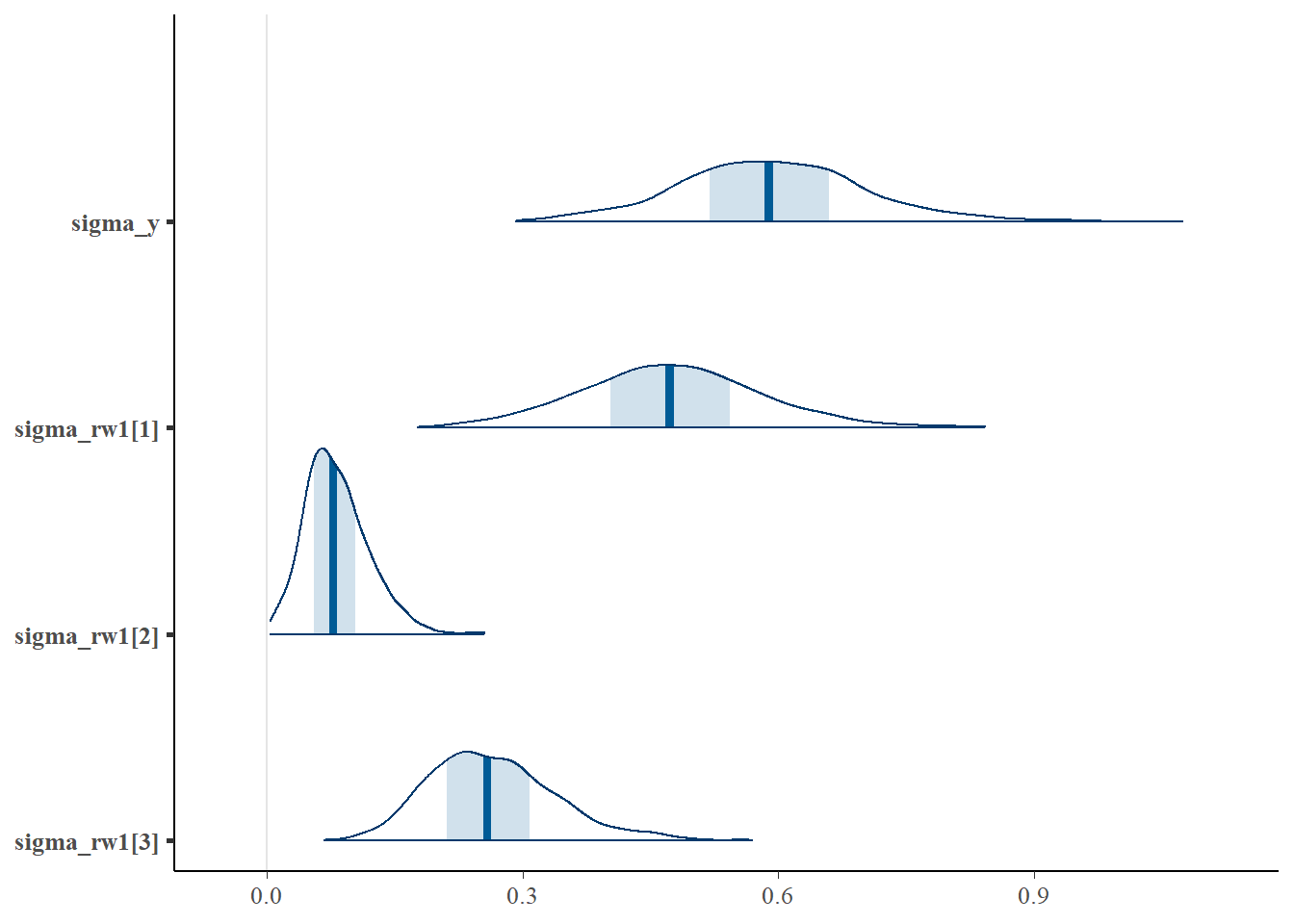Let’s check how well our estimates of $$\beta$$ coincide with the true values (the solid lines correspond to true values):

betas <- summary(fit$stanfit, "beta_rw")$summary[, "mean"]

ts.plot(cbind(rw, beta1, beta2, matrix(betas, ncol = 3)),
col = rep(1:3, 2), lty = rep(1:2, each = 3))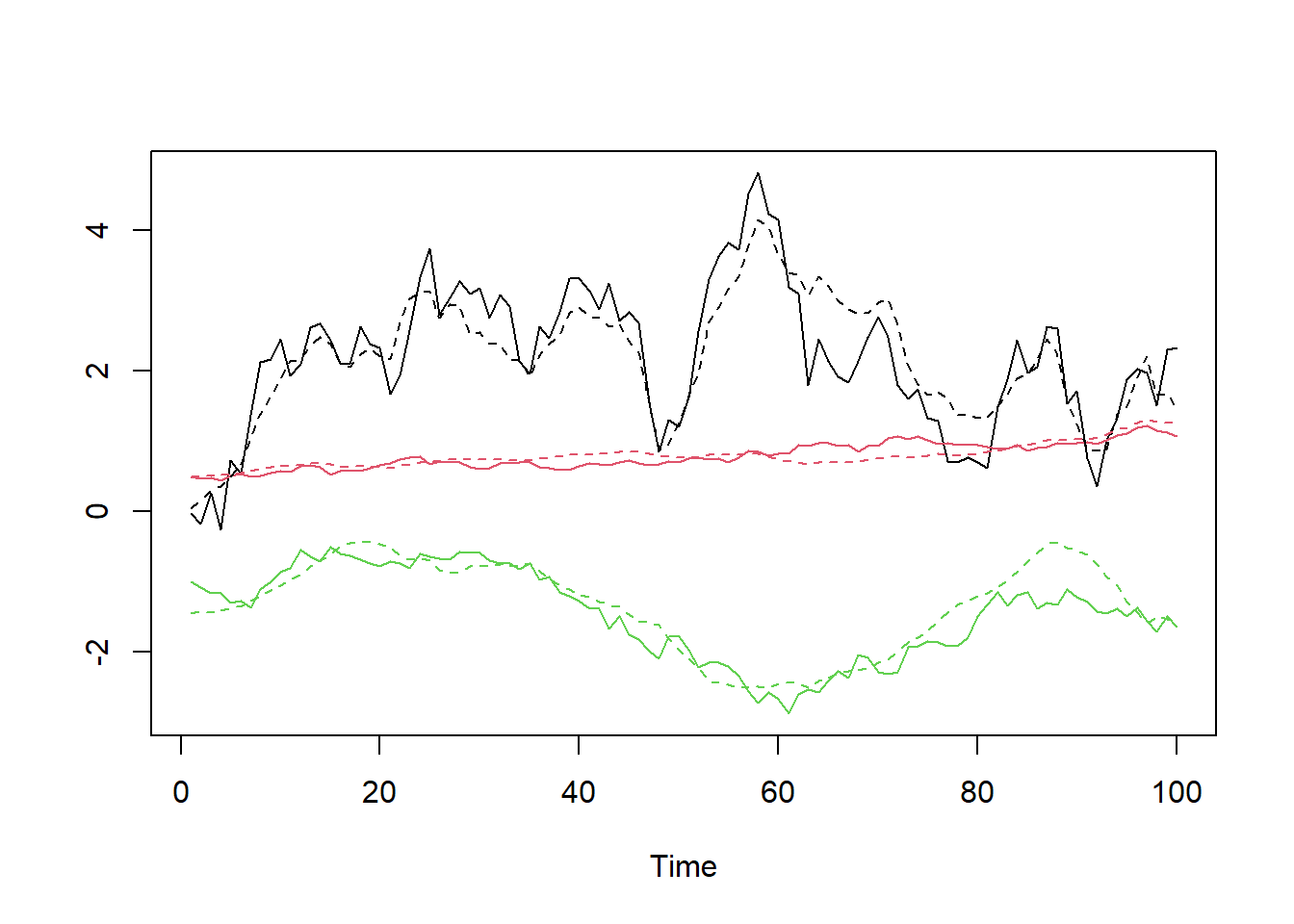There is also simpler (and prettier) ggplot2 based plotting function for coefficients:

plot_coefs(fit, scales = "free") + ggplot2::theme_bw()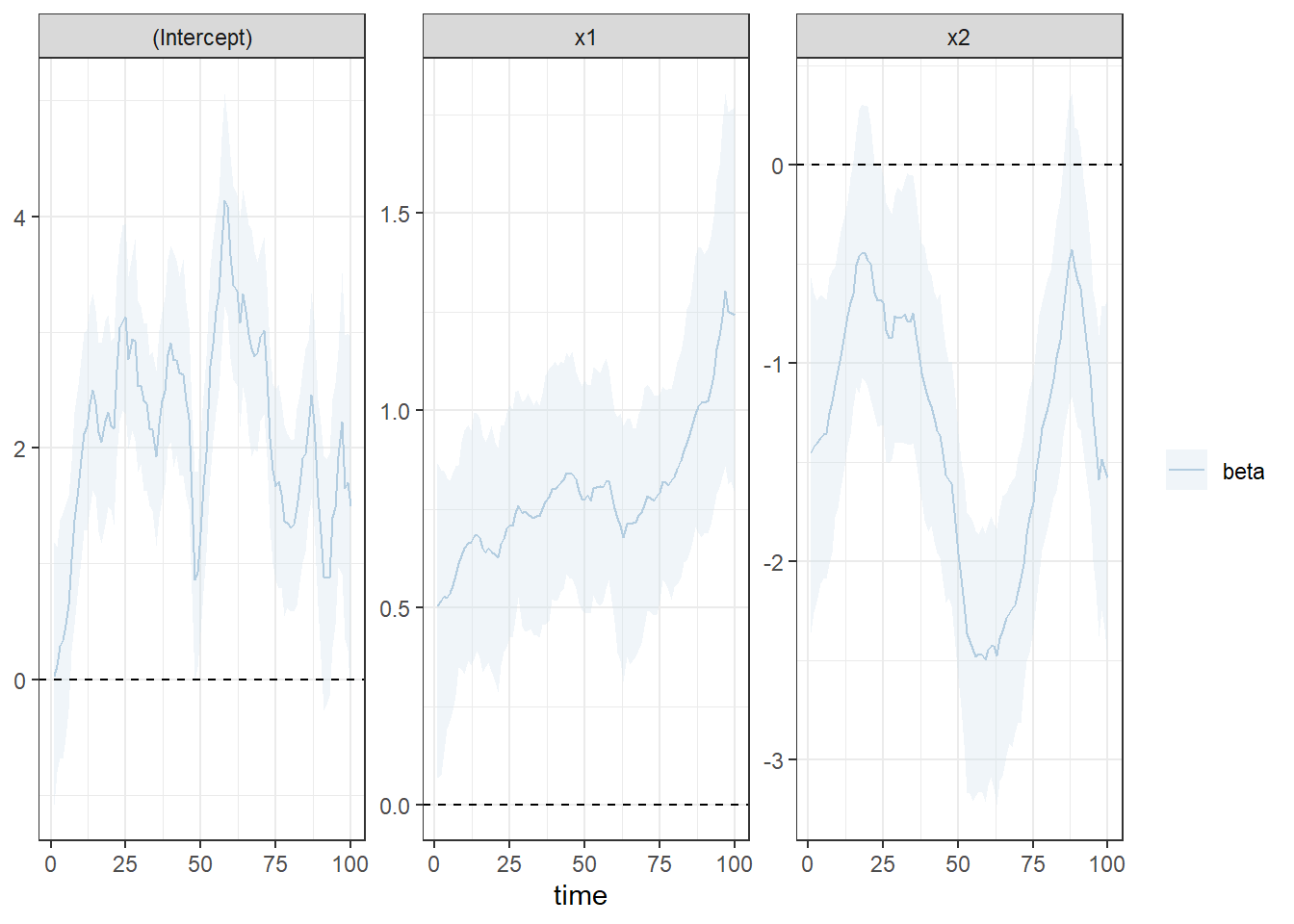### Posterior predictive checks

The walker function also returns samples from the posterior predictive distribution $$p(y^{\textrm{rep}} | y) = \int p(y^{\textrm{rep}} | \beta, \sigma, y) p(\beta, \sigma | y) \textrm{d}\beta\textrm{d}\sigma$$. This can be used to used for example in assessment of model fit to the data. By comparing the replication series (mean and 95% quantiles in black) and the original observations (in red) we see that very good overlap, which is not that surprising given that we know the correct model:

pp_check(fit)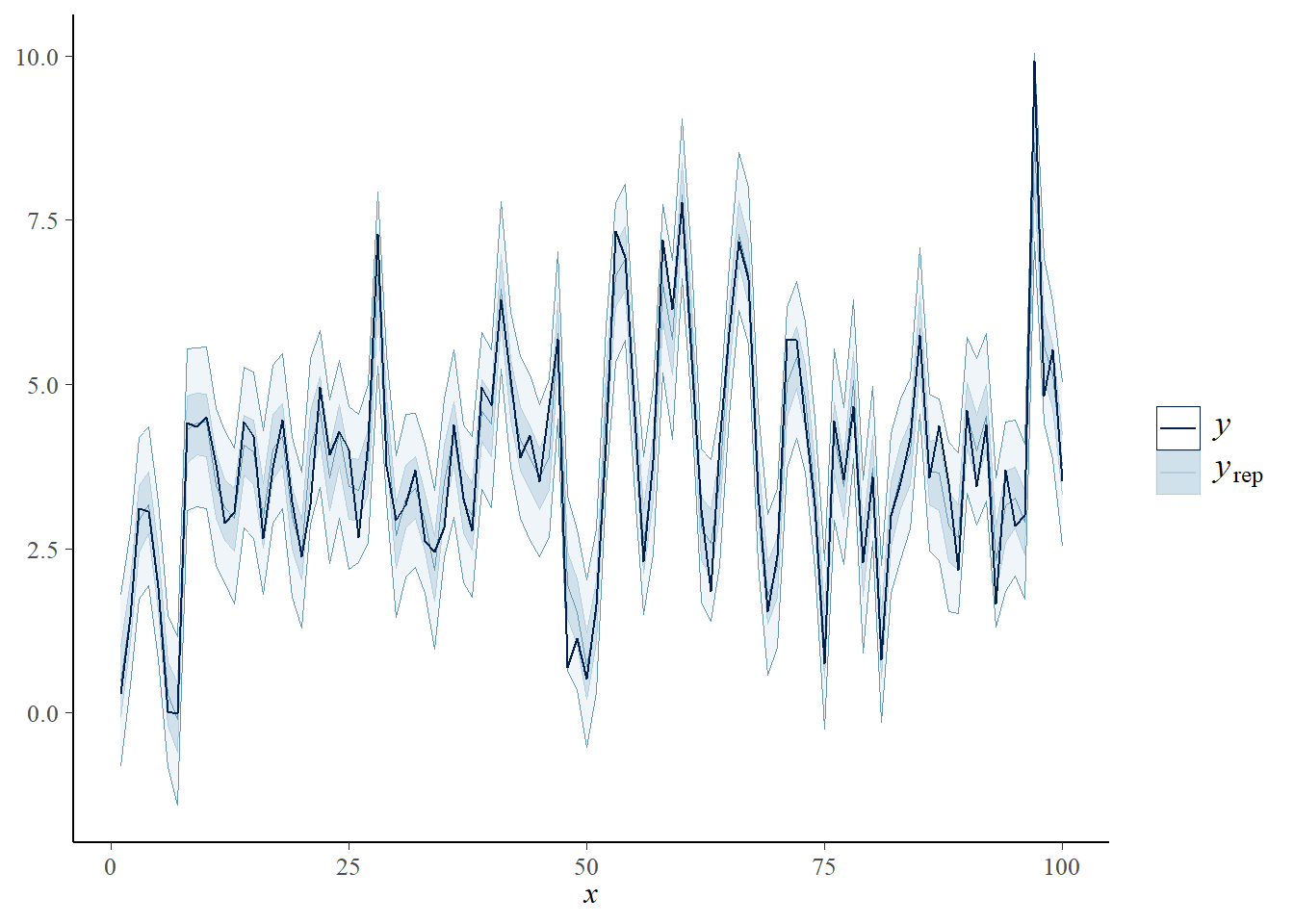It is also possible to perform leave-future-out cross validation (LFO-CV) for the walker models. While the exact LFO-CV can be computationally demanding, walker also supports approximate PSIS-LFO-CV via function lfo.

### Counterfactual predictions

We can also produce “counterfactual” predictions using our estimated model. For example, we can ask what if x1 variable was constant 0 from time point 60 onward:

library(ggplot2)
## Warning: package 'ggplot2' was built under R version 4.3.1
library(dplyr)
##
## Attaching package: 'dplyr'
## The following objects are masked from 'package:stats':
##
##     filter, lag
## The following objects are masked from 'package:base':
##
##     intersect, setdiff, setequal, union
fitted <- fitted(fit) # estimates given our actual observed data
newdata <- data.frame(x1 = c(x1[1:59], rep(0, 41)), x2 = x2)
pred_x1_1 <- predict_counterfactual(fit, newdata, type = "mean")

cbind(as.data.frame(rbind(fitted, pred_x1_1)),
type = rep(c("observed", "counterfactual"), each = n), time = 1:n) %>%
ggplot(aes(x = time, y = mean)) +
geom_ribbon(aes(ymin = 2.5%, ymax = 97.5%, fill = type), alpha = 0.2) +
geom_line(aes(colour = type)) + theme_bw()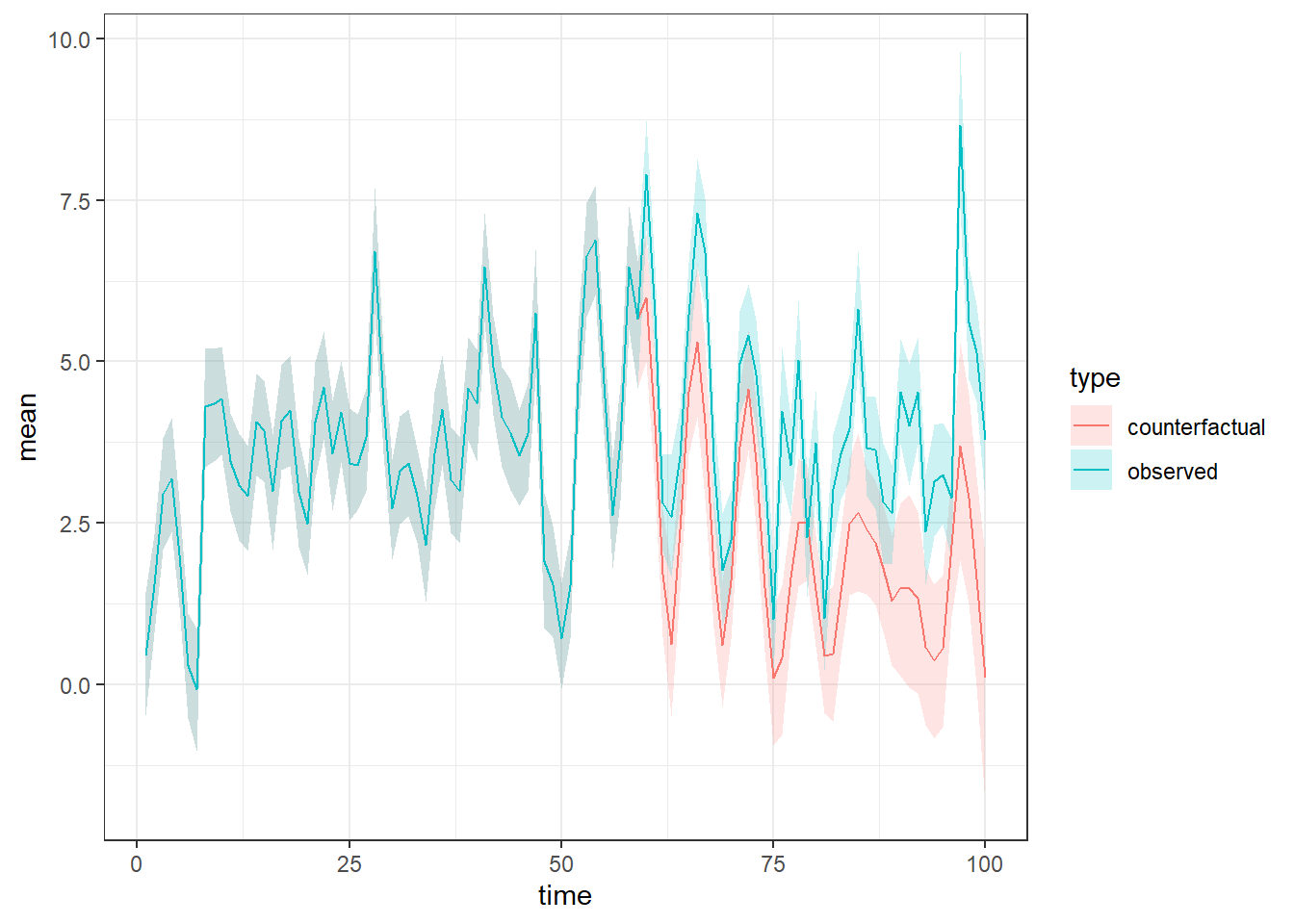### Out-of-sample prediction

It is also possible to obtain actual forecasts given new covariates $$x^{new}$$:

new_data <- data.frame(x1 = rnorm(10, mean = 2), x2 = cos((n + 1):(n + 10)))
pred <- predict(fit, new_data)
plot_predict(pred)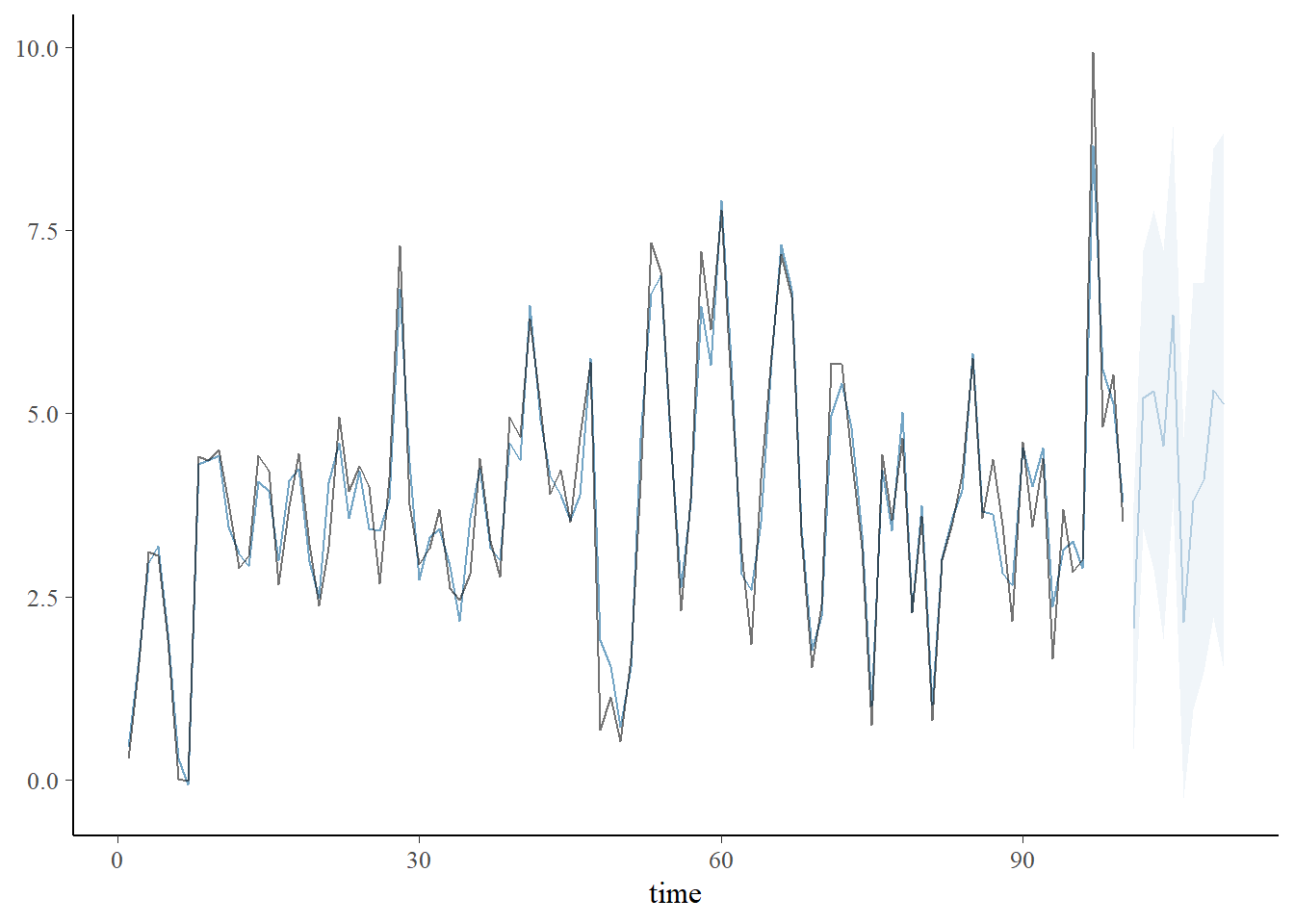## Extensions: Smoother effects and non-Gaussian models

When modelling regression coefficients as a simple random walk, the posterior estimates of these coefficients can have large short-term variation which might not be realistic in practice. One way of imposing more smoothness for the estimates is to switch from random walk coefficients to integrated second order random walk coefficients: $\beta_{t+1} = \beta_t + \nu_t,\\ \nu_{t+1} = \nu_t + \eta_t.$ This is essentially local linear trend model with restriction that there is no noise on the $$\beta$$ level. This model can be estimated by switching rw1 function inside of the walker formula to rw2, with almost identical interface, but now $$\sigma$$ correspond to the standard deviations of the slope terms $$\nu$$. The Gaussian prior for $$\nu_1$$ most also be defined. Using RW2 model, the coefficient estimates of our example model are clearly smoother:

fit_rw2 <-walker(y ~ -1 +
rw2(~ x1 + x2, beta = c(0, 10), sigma = c(2, 0.001), nu = c(0, 10)),
refresh = 0, init = 0, chains = 1, sigma_y = c(2, 0.001))
plot_coefs(fit_rw2, scales = "free") + ggplot2::theme_bw()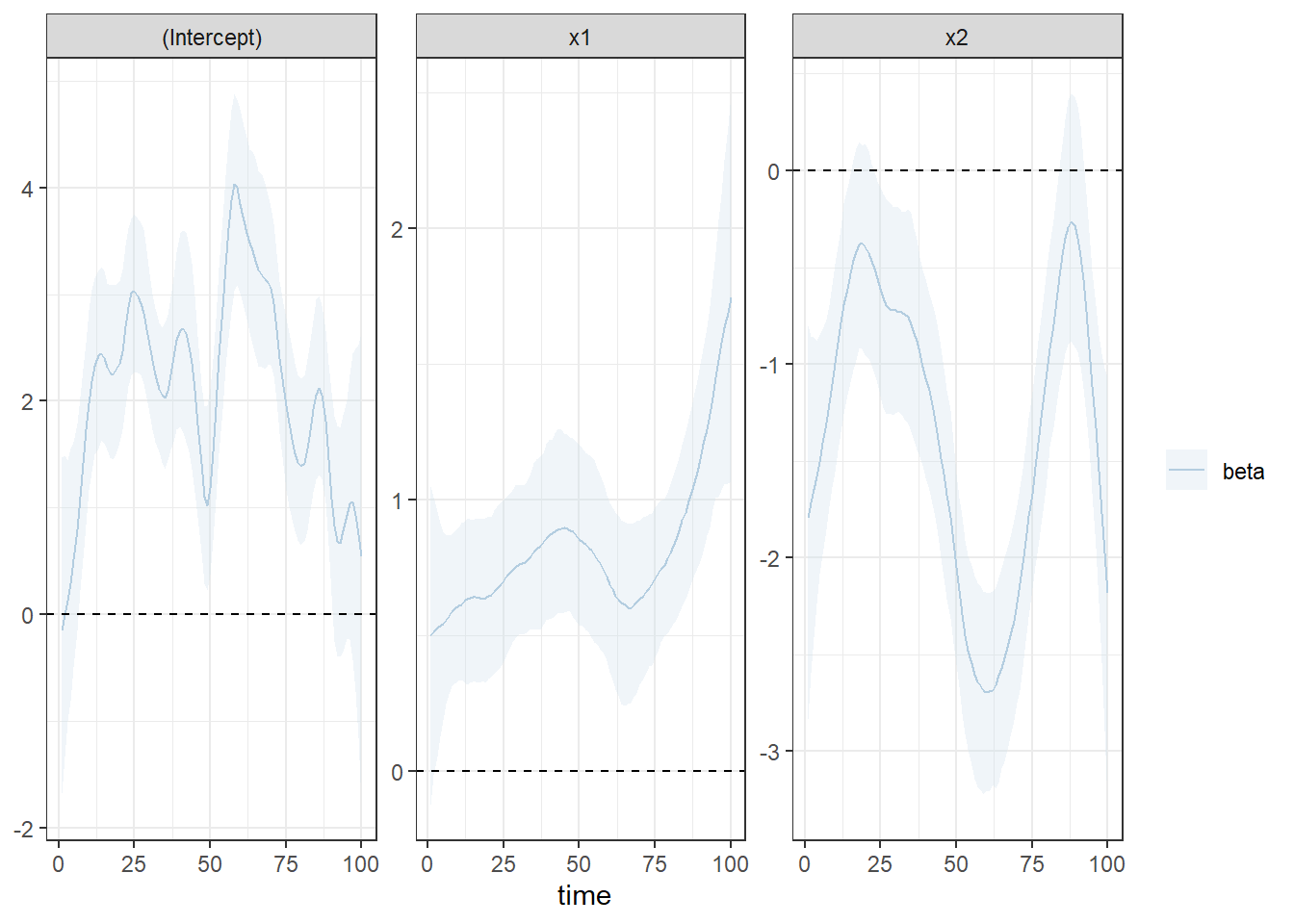So far we have focused on simple Gaussian linear regression. The above treatment cannot be directly extended to non-Gaussian models such as Poisson regression, as the marginal log-likelihood is intractable. However, it is possible to use relatively accurate Gaussian approximations, and the resulting approximate posterior can then be weighted using importance sampling type correction , leading to asymptotically exact inference (if necessary). Function walker_glm extends the the package to handle Poisson and binomial observations using the aforementioned methodology. Further extension to negative binomial distribution is planned in future.

## Comparison with naive approach

We now compare the efficiency of the “naive” implementation and the state space approach. For this, we use function walker_rw1, which supports only basic random walk models (here priors for standard deviations are defined as truncated normal distributions). We can perform the same analysis with naive implementation by setting the argument naive to TRUE. Note that in this case we need to adjust the default sampling parameters (argument control) to avoid (most of the) problems in sampling.

set.seed(1) # set seed to simulate same initial values for both methods
naive_fit <- walker_rw1(y ~ x1 + x2, refresh = 0,
chains = 2, cores = 2, iter = 1e4,
beta = cbind(0, rep(5, 3)), sigma = cbind(0, rep(2, 4)),
naive = TRUE,
control = list(adapt_delta = 0.999, max_treedepth = 12))

set.seed(1)
kalman_fit <- walker_rw1(y ~ x1 + x2, refresh = 0,
chains = 2, cores = 2, iter = 1e4,
beta = cbind(0, rep(5, 3)), sigma = cbind(0, rep(2, 4)),
naive = FALSE)

(In order to keep CRAN checks fast, the results here are precomputed.)

With naive implementation we get some warnings and much higher computation time:

check_hmc_diagnostics(naive_fit$stanfit) ## ## Divergences: ## 14 of 10000 iterations ended with a divergence (0.14%). ## Try increasing 'adapt_delta' to remove the divergences. ## ## Tree depth: ## 0 of 10000 iterations saturated the maximum tree depth of 12. ## ## Energy: ## E-BFMI indicated no pathological behavior. check_hmc_diagnostics(kalman_fit$stanfit)
##
## Divergences:
## 0 of 10000 iterations ended with a divergence.
##
## Tree depth:
## 0 of 10000 iterations saturated the maximum tree depth of 10.
##
## Energy:
## E-BFMI indicated no pathological behavior.
get_elapsed_time(naive_fit$stanfit) ## warmup sample ## chain:1 716.958 416.457 ## chain:2 761.639 409.833 get_elapsed_time(kalman_fit$stanfit)
##         warmup sample
## chain:1 21.213 23.804
## chain:2 20.374 24.557

Naive implementation produces also much smaller effective sample sizes:

print(naive_fit$stanfit, pars = c("sigma_y", "sigma_b")) ## Inference for Stan model: rw1_model_naive. ## 2 chains, each with iter=10000; warmup=5000; thin=1; ## post-warmup draws per chain=5000, total post-warmup draws=10000. ## ## mean se_mean sd 2.5% 25% 50% 75% 97.5% n_eff Rhat ## sigma_y 0.51 0.01 0.13 0.23 0.43 0.52 0.60 0.75 315 1 ## sigma_b 0.59 0.01 0.13 0.36 0.50 0.58 0.67 0.85 579 1 ## sigma_b 0.08 0.00 0.04 0.01 0.05 0.07 0.10 0.17 1335 1 ## sigma_b 0.31 0.00 0.10 0.15 0.24 0.30 0.37 0.54 2162 1 ## ## Samples were drawn using NUTS(diag_e) at Thu Jan 27 16:03:57 2022. ## For each parameter, n_eff is a crude measure of effective sample size, ## and Rhat is the potential scale reduction factor on split chains (at ## convergence, Rhat=1). print(kalman_fit$stanfit, pars = c("sigma_y", "sigma_b"))
## Inference for Stan model: rw1_model.
## 2 chains, each with iter=10000; warmup=5000; thin=1;
## post-warmup draws per chain=5000, total post-warmup draws=10000.
##
##            mean se_mean   sd 2.5%  25%  50%  75% 97.5% n_eff Rhat
## sigma_y    0.49       0 0.14 0.14 0.41 0.50 0.58  0.74  2417    1
## sigma_b 0.60       0 0.13 0.36 0.51 0.59 0.68  0.88  3837    1
## sigma_b 0.08       0 0.04 0.01 0.05 0.08 0.10  0.17  5404    1
## sigma_b 0.31       0 0.10 0.15 0.24 0.30 0.37  0.55  6033    1
##
## Samples were drawn using NUTS(diag_e) at Thu Jan 27 16:04:48 2022.
## For each parameter, n_eff is a crude measure of effective sample size,
## and Rhat is the potential scale reduction factor on split chains (at
## convergence, Rhat=1).

## Discussion

In this vignette we illustrated the benefits of marginalisation in the context of time-varying regression models. The underlying idea is not new; this approach is typical in classic Metropolis-type algorithms for linear-Gaussian state space models where the marginal likelihood $$p(y | \theta)$$ (where $$\theta$$ denotes the hyperparameters i.e. not the latents states such as $$\beta$$’s in current context) is used in the computation of the acceptance probability. Here we rely on readily available Hamiltonian Monte Carlo based Stan software, thus allowing us to enjoy the benefits of diverse tools of the Stan community.

The original motivation behind the walker was to test the efficiency of importance sampling type weighting method of Vihola, Helske, and Franks (2020) in a dynamic generalized linear model setting within the HMC context. Although some of our other preliminary results have suggested that naive combination of the HMC with IS weighting can provide rather limited computational gains, in case of GLMs with time-varying coefficients so called global approximation technique provides fast and robust alternative to standard HMC approach of Stan.

## Acknowledgements

This work has been supported by the Academy of Finland research grants 284513, 312605, 311877, and 331817.